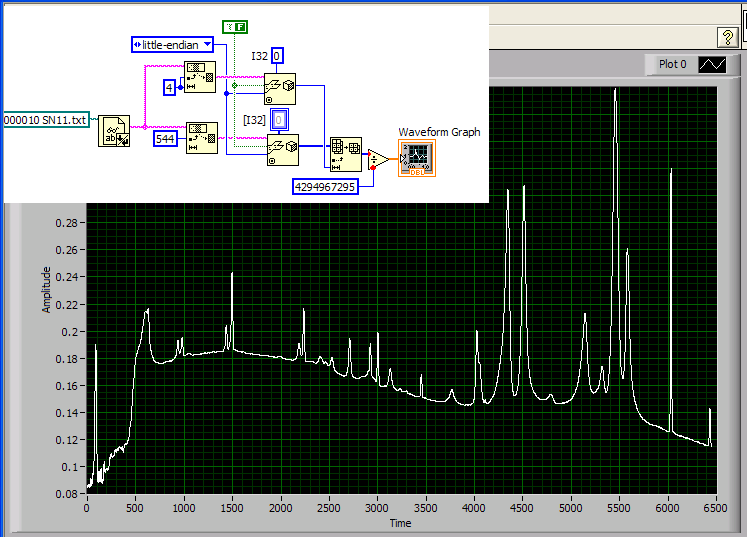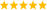# LabVIEW

cancel
Showing results for
Did you mean:

Highlighted

## Interpreting values supposedly in fixed-point format

Hi - I am close to being able to open a Thermo Scientific Industries (formerly Galactic) SPC file just using LabVIEW but the data values are stored in a strange fashion (versus just pure floating point).  Here is the documentation from the developer and the company is no longer supporting this format and will not answer questions about it.  I wanted to know if someone can shed some light on this and save me some time.

* Data Value Formats
* ==================
* Y values are represented as fixed-point signed fractions (which are similar
* to integers except that the binary point is above the most significant bit
* rather than below the least significant) scaled by a single exponent value.
* For example, 0x40000000 represents 0.25 and 0xC0000000 represents -0.25 and
* if the exponent is 2 then they represent 1 and -1 respectively. Note that
* in the old 0x4D format, the two words in a 4-byte DP Y value are reversed.
* To convert the fixed Y values to floating point:
* FloatY = (2^Exponent)*FractionY

I can read the header from the format spec in the documentatino. I obtained the exponent value from the header.  I just don't really understand how to read in the data values, and I am not sure I understand the example the developer gives regarding "0x40000000 represents 0.25 and 0xC0000000 represents -0.25 and
* if the exponent is 2 then they represent 1 and -1 respectively."

Header appears to be 512 bytes long looking at this file in a Hex editor (the other header length options were 224 and 246 depending on the file age).

Sincerely,

Don

Message Edited by DonRoth on 05-23-2008 02:17 PM
Message 1 of 34
(1,474 Views)

## Re: Interpreting values supposedly in fixed-point format

ps. I know what the Y values are supposed to be - they are those in the second (B) column of the attached .xls file.
Message 2 of 34
(1,455 Views)

## Re: Interpreting values supposedly in fixed-point format

Don,

I think this is what the developer did.

Think of it as a 32 bit binary number with an imaginary binary point to the far left.  Left to right - 1 sign bit, 31 bits for fraction.  MSB - LSB

For the case of 0x40000000    Sign bit is zero so it is a positive number.  fracton = 1*(2^-2) = 0.25

For the case of 0xC0000000   Sign bit is one so it is a negative number.  fracton = -1*(2^-2) = -0.25

For the case of 0x70000000  fraction = 1 * (2^-2) + (2^-3) + (2^-4) = .4375  ( If I can still run my calculator right !)

If I remember correctly, LV8.5 supports fixed-point data types to deal with this kind of stuff.

Message 3 of 34
(1,443 Views)

## Re: Interpreting values supposedly in fixed-point format

I don't quite get where the "exponent" comes from. Is this the same for the entire datafile?

Anyway, to get the data, you need to unflatten it from string as little endian I32 and then divide by 2^32 and multiply by the exponent.

File position 4 has a 4 byte number that seems to represent the data size in # of points (6453 in your case). The data starts at 544.

There is a lot of other information in the file, I am sure the exponent is somewhere. You need the full file specification.

Here's a quick attempt at reading the file assuming an exponent of 1. See if it get's you any closer. Good luck! 🙂

(the code is very crude. Typically  you would want to take the subset with length 4x6456 before unflattening the data.)Message Edited by altenbach on 05-23-2008 02:45 PMLabVIEW Champion. It all comes together inGCentralMessage 4 of 34
(1,438 Views)

## Re: Interpreting values supposedly in fixed-point format

Altenbach - that is indeed what the data looks like but it needs to be scaled properly.  The exponent is read in as CHAR (I was only able to read it in using U8) at byte offset position 3.   I get value of 14.  Then we still need to know how to read in the data per the fixed point numbers and extract the bits.

Centerbolt - I don't understand your math.  You have 1*(2^2) = 0.25?  Yes, LabVIEW does fixed point but I am not sure how to extract the bits from the fixed point number using LabVIEW functionality in order to do the calculation.  So the examples the developer gives are still not clear.

Sincerely,

Don

Message Edited by DonRoth on 05-27-2008 11:09 AM
Message 5 of 34
(1,381 Views)

## Re: Interpreting values supposedly in fixed-point format

DonRoth wrote:
I get value of 14.  Then we still need to know how to read in the data per the fixed point numbers and extract the bits.

Unless the multiplier is an integer power of two, you cannot directly cast it as fixed point type. Use my code to read it and multiply the DBL result by the multiplier.

Do you really need the result in FXP or is DBL sufficient? The problem is that the FXP representation probably needs to be adjusted depending on the multiplier value else you'll possibly discard bits.LabVIEW Champion. It all comes together inGCentralMessage 6 of 34
(1,372 Views)

## Re: Interpreting values supposedly in fixed-point format

DonRoth wrote:
I get value of 14.
Could it be the multiplier is 2^14 instead? Would make more sense.LabVIEW Champion. It all comes together inGCentralMessage 7 of 34
(1,370 Views)

## Re: Interpreting values supposedly in fixed-point format

2 raised to the (-2) power = 0.25
Message 8 of 34
(1,368 Views)

## Re: Interpreting values supposedly in fixed-point format

No, the unscaled values are -0.25...0.25, which then need to be multiplied by the multiplier for the final result, which is no longer in this range unless the multipler is 1.LabVIEW Champion. It all comes together inGCentralMessage 9 of 34
(1,365 Views)

## Re: Interpreting values supposedly in fixed-point format

Altenbach,

All I attempted to explain was how the floating point version of the fraction Y was arrived at.

* Data Value Formats
* ==================
* Y values are represented as fixed-point signed fractions (which are similar
* to integers except that the binary point is above the most significant bit
* rather than below the least significant) scaled by a single exponent value.
* For example, 0x40000000 represents 0.25 and 0xC0000000 represents -0.25 and
* if the exponent is 2 then they represent 1 and -1 respectively. Note that
* in the old 0x4D format, the two words in a 4-byte DP Y value are reversed.
* To convert the fixed Y values to floating point:
* FloatY = (2^Exponent)*FractionY

I'm fully aware that the overall conversion is not complete without the scaling factor ( 2^Exponent ).

Message 10 of 34
(1,354 Views)